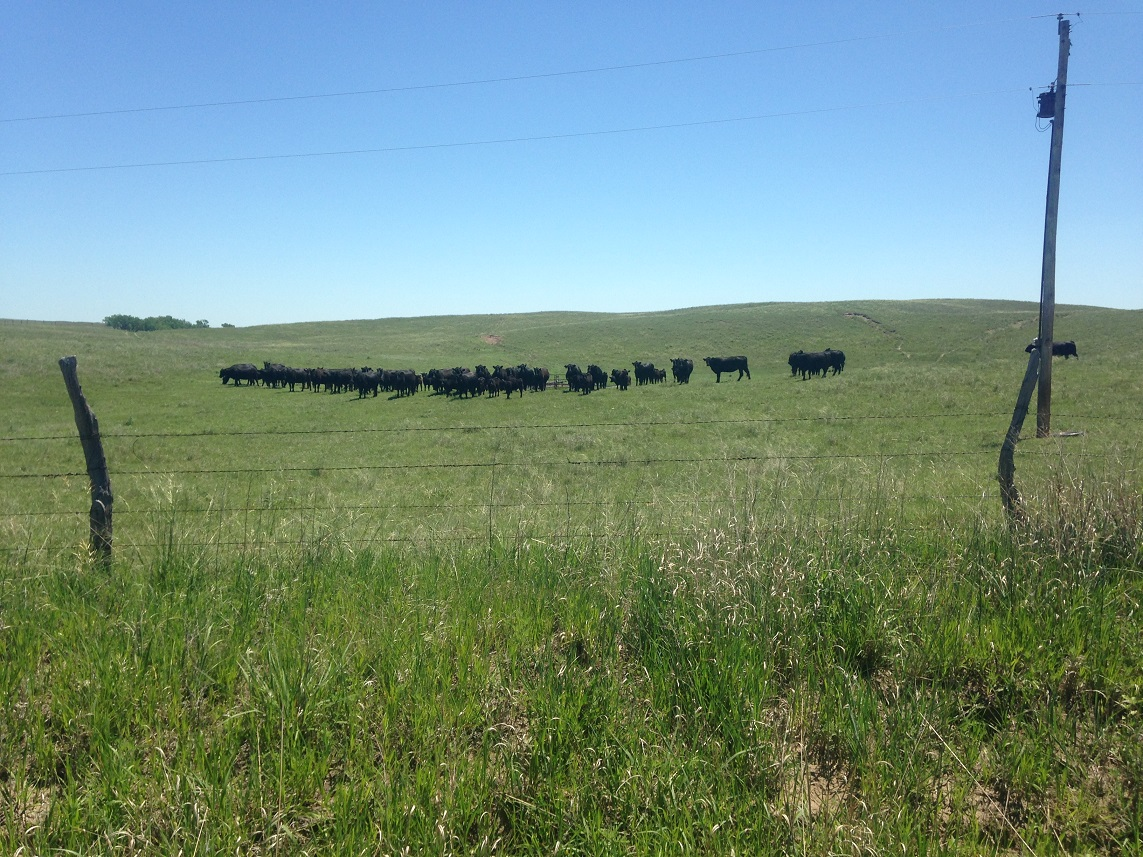# Measuring Your Cost of Production and Profit MarginIn general, the Unit Cost of Production (UCOP) is simply your Total Costs divided by Total Production. Photo courtesy of Jay Parsons.

By Jay Parsons, Department of Agricultural Economics, University of Nebraska-Lincoln

The cost of producing one unit of output is extremely important information in any business. It determines your break-even price which, in turn, helps determine pricing decisions and profit margins. It also provides a baseline for comparison to other producers with the same or similar output so you can see how competitive you are at producing the product in question.

In general, the Unit Cost of Production (UCOP) is simply your Total Costs divided by Total Production. For a business selling a single output, it doesn’t need to get more complicated than this as long as you properly account for all of your costs and accurately measure your production. Unfortunately, most farms and ranches are not quite this simple and it becomes necessary to break down costs and revenues on an enterprise basis to get a handle on your UCOP for any one output.

However, suppose you are a cow/calf producer selling weaned calves in the fall each year. In your mind, this is your product. Your annual calf sales total to 920 cwt. sold generating revenue of \$200,376 for an average price of \$217.80 per cwt.

Suppose, your annual costs total to \$217,984 when you fully account for everything except for owner labor. Dividing \$217,984 by 920 would yield a UCOP of \$236.94 per cwt. However, this is not an accurate cost measure to use to calculate your profit margin compared to the average sales price of \$217.80. This is because you sold more than just calves during the year. You also sold some cull animals. How do we deal with this issue? There are three standard methods to consider.

Let’s start by assuming your annual cull revenue totals \$60,560.

METHOD 1: PER UNIT SOLD METHOD
You can compare income per unit sold rather than the calf price to the UCOP to determine your profit margin. In this case, total income from calf sales and cull sales is \$200,376 + \$60,560 = \$260,936. Dividing this total income of \$260,936 by the 920 cwt. of calves sold results in \$283.63 in income per cwt. of calf sold. Compared to the UCOP of \$236.94, this implies a profit margin of \$46.69 per cwt.

METHOD 2: RESIDUAL COSTS METHOD
You can subtract your cull revenue from your total costs to arrive at a residual cost value that can be compared to sales price. In this case, your total costs of \$217,984 minus cull revenue of \$60,560 results in residual costs of \$157,424. Dividing residual costs of \$157,424 by 920 cwt. sold results in a UCOP of \$171.11 per cwt. Compared to the selling price of \$217.80, the profit margin would again be \$46.69 per cwt.

METHOD 3: EQUIVALENT PRODUCTION METHOD
You can use your total income and average selling price to calculate an equivalent number of units sold and use that to calculate your UCOP instead of the actual number of units sold. In this case, you would divide your total income from calves and culls of \$260,936 by the average selling price of calves of \$217.80 to calculate an equivalent sales volume of 1,198 cwt. Dividing your total costs of \$217,984 by this equivalent sales volume of 1,198 cwt. results in a UCOP of \$181.95 per cwt. Compared to a selling price of \$217.80 per cwt., this would imply a profit margin of \$35.85 per cwt. for 1,198 cwt. of equivalent production.

SUMMARY
So, which method is better? It depends upon your perspective but you need to make sure you keep track of the assumptions behind the scenes.

Method 1 attributes all of the costs and all of the revenue to the actual units (cwt.) of calves sold. You compare the UCOP to the total income generated per unit sold rather than the actual selling price. The profit margin of \$46.69 per cwt. is entirely attributed to the actual calf sales of 920 cwt. which is to say that no profit is attributed to the cull sales. Total profit would be \$46.69 per cwt. times 920 cwt. or \$42,955.

Method 2 gives you a UCOP of \$171.11 per cwt. that you can compare directly to the actual selling price of \$217.80 per cwt. It also assumes that cull sales are a break-even enterprise and the entire \$46.69 per cwt. profit margin is attributed to the actual calf sales of 920 cwt.

Method 3 also gives you a UCOP (\$181.95 per cwt.) that you can compare directly to the actual selling price of \$217.80 per cwt. but, unlike the other two methods, Method 3 assumes that cull sales have an equivalent profit margin percentage as the calf sales. The profit margin of \$35.85 per cwt. is applied to 920 cwt. of actual calf sales and 278 cwt. of equivalent cull sales. This implies that the \$42,955 in profit is split between the calf enterprise (\$32,982) and the cull enterprise (\$9,973).

Some people are most comfortable with Method 3. However, other folks prefer to think of their cull sales as a break-even proposition and would opt for Method 1 or 2. The method you use is less important than understanding your result and how to compare it to the price you are getting.

Jay Parsons
Farm and Ranch Management Specialist
Department of Agricultural Economics